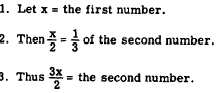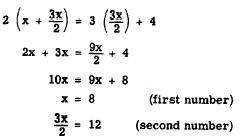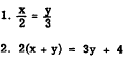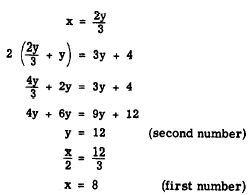Using two variables in solving word problemsCustom SearchUSING TWO VARIABLES IN SOLVING WORD PROBLEMS Many problems can be solved quickly and easily using one equation with one variable. Other problems that might be rather difficult to solve in terms of one variable can easily be solved using two equations and two variables. The difference in the two methods is shown in the following example, solved first by using one variable and then using two. EXAMPLE: Find the two numbers such that half the first equals a third of the second and twice their sum exceeds three times the second by 4. SOLUTION USING ONE VARIABLE:From the statement of the problem, we then haveSOLUTION USING TWO VARIABLES: If we let x and y be the first and second numbers, respectively, we can write two equations almost directly from the statement of the problem. Thus,Solving for x in the first equation and substituting this value in the second, we haveThus, we see that the solution using two variables is more direct and simple. Often it would require a great deal of skill to manipulate a problem so that it might be solved using one variable; whereas the solution using two variables might be very simple. The use of two variables, of course, involves the fact that the student must be able to form two equations from the information given in the problem. Practice problems. Solve the following problems using two variables: 1. A Navy tug averages 12 miles per hour downstream and 9 miles per hour upstream. How fast is the stream flowing? 2. The sum of the ages of two boys is 18. If 4 times the younger boy’s age is subtracted from 3 times the older boy’s age, the difference is 12. What are the ages of the two boys? Answers: 1. 1 1/2 mph. 2. 6 years and 12 years. INEQUALITIES IN TWO VARIABLES Inequalities in two variables are of the following form:         x+y>2 Many solutions of such an inequation are apparent immediately. For example, x could have the value 2 and y could have the value 3, since 2 + 3 is greater than 2. The existence of a large number of solutions suggests that a graph of the inequation would contain many points. The graph of an inequation in two unknowns is, in fact, an entire area rather than just a line.Integrated Publishing, Inc. - A (SDVOSB) Service Disabled Veteran Owned Small Business